# Coplanarity of Two Lines in 3D Geometry

Given two lines L1 and L2, each passing through a point whose position vector are given as (X, Y, Z) and parallel to line whose direction ratios are given as (a, b, c), the task is to check whether line L1 and L2 are coplanar or not.

Coplanar: If two lines are in a same plane then lines can be called as coplanar.

Example:

Input:
L1: (x1, y1, z1) = (-3, 1, 5) and (a1, b1, c1) = (-3, 1, 5)
L2: (x1, y1, z1) = (-1, 2, 5) and (a1, b1, c1) = (-1, 2, 5)
Output: Lines are Coplanar

Input:
L1: (x1, y1, z1) = (1, 2, 3) and (a1, b1, c1) = (2, 4, 6)
L2: (x1, y1, z1) = (-1, -2, -3) and (a1, b1, c1) = (3, 4, 5)
Output: Lines are Non-Coplanar

Approach: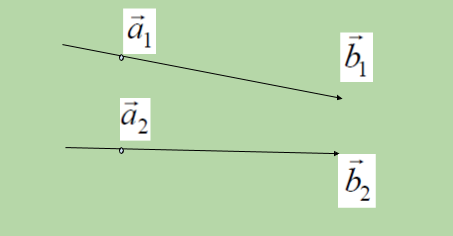There are two ways to express a line in 3 dimension:

Vector Form:

The equation of two lines whose coplanarity is to be determined in vector form.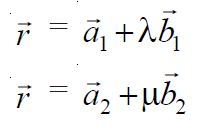In above equation of line a vector is the point in 3D plane from which given line is passing through called as position vector a and b vector is the vector line in 3D plane to which our given line is parallel. So it can be said that the line(1) passes through the point, say A, with position vector a1 and is parallel to vector b1 and the line(2) passes through the point, say B with position vector a2 and is parallel to vector b2. Therefore: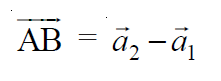The given lines are coplanar if and only if AB vector is perpendicular  to cross product of vectors b1 and b2 i.e.,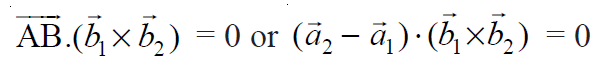Here cross product of vectors b1 and b2 will give the another vector line which will be perpendicular to both b1 and b2 vector lines. and AB is the line vector joining the position vectors a1 and a2 of two given lines.  Now, check whether two lines are coplanar or not by determining above dot product is zero or not.

Cartesian Form:

Let (x1, y1, z1) and (x2, y2, z2) be the coordinates of the points A and B respectively.
Let a1, b1, c1 and a2, b2, c2 be the direction ratios of vectors b1 and  b2 respectively. Then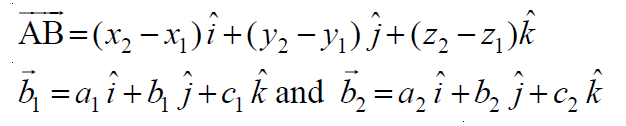The given lines are coplanar if and only if: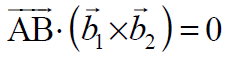In Cartesian Form it can be expressed as: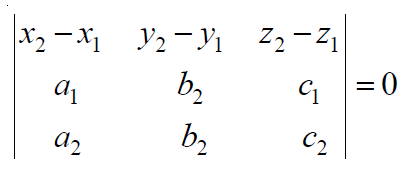Therefore, for both type of forms needs a position vectors a1 and a2 in input as (x1, y1, z1) and (x2, y2, z2) respectively and direction ratios of vectors b1 and b2 as (a1, b1, c1) and (a2, b2, c2) respectively.

Follow the steps below to solve the problem:

• Initialize a 3 X 3 matrix to store the elements of the Determinant shown above.
• Calculate the cross product of b2 and b1 and dot product of (a2 – a1).
• If the value of the Determinant is 0, the lines are coplanar. Otherwise, they are non-coplanar.

Below is the implementation of the above approach:

## Python3

 `# Python Program implement ` `# the above approach ` ` `  `# Function to generate determinant ` `def` `det(d): ` `    ``Sum` `=` `d[``0``][``0``] ``*` `((d[``1``][``1``] ``*` `d[``2``][``2``])  ` `                    ``-` `(d[``2``][``1``] ``*` `d[``1``][``2``])) ` `    ``Sum` `-``=` `d[``0``][``1``] ``*` `((d[``1``][``0``] ``*` `d[``2``][``2``])  ` `                    ``-` `(d[``1``][``2``] ``*` `d[``2``][``0``])) ` `    ``Sum` `+``=` `d[``0``][``2``] ``*` `((d[``0``][``1``] ``*` `d[``1``][``2``])  ` `                    ``-` `(d[``0``][``2``] ``*` `d[``1``][``1``])) ` ` `  `    ``# Return the sum ` `    ``return` `Sum` ` `  `# Driver Code ` `if` `__name__ ``=``=` `'__main__'``: ` ` `  `    ``# Position vector of first line ` `    ``x1, y1, z1 ``=` `-``3``, ``1``, ``5` ` `  `    ``# Direction ratios of line to ` `    ``# which first line is parallel ` `    ``a1, b1, c1 ``=` `-``3``, ``1``, ``5` ` `  `    ``# Position vectors of second line ` `    ``x2, y2, z2 ``=` `-``1``, ``2``, ``5` ` `  `    ``# Direction ratios of line to ` `    ``# which second line is parallel ` `    ``a2, b2, c2 ``=` `-``1``, ``2``, ``5` ` `  `    ``# Determinant to check coplanarity  ` `    ``det_list ``=` `[[x2``-``x1, y2``-``y1, z2``-``z1],  ` `                ``[a1, b1, c1], [a2, b2, c2]] ` ` `  `    ``# If determinant is zero ` `    ``if``(det(det_list) ``=``=` `0``): ` `        ``print``(``"Lines are coplanar"``) ` ` `  `    ``# Otherwise ` `    ``else``: ` `        ``print``(``"Lines are non coplanar"``) `

Output:

```Lines are coplanar
```

Time Complexity: O(1)
Auxiliary Space: O(1)

Attention reader! Don’t stop learning now. Get hold of all the important DSA concepts with the DSA Self Paced Course at a student-friendly price and become industry ready.

My Personal Notes arrow_drop_upCheck out this Author's contributed articles.

If you like GeeksforGeeks and would like to contribute, you can also write an article using contribute.geeksforgeeks.org or mail your article to contribute@geeksforgeeks.org. See your article appearing on the GeeksforGeeks main page and help other Geeks.

Please Improve this article if you find anything incorrect by clicking on the "Improve Article" button below.